# GSEB Solutions Class 11 Chemistry Chapter 5 States of Matter

Gujarat Board GSEB Textbook Solutions Class 11 Chemistry Chapter 5 States of Matter Textbook Questions and Answers.

## Gujarat Board Textbook Solutions Class 11 Chemistry Chapter 5 States of Matter

### GSEB Class 11 Chemistry States of Matter Text Book Questions and AnswersQuestion 1.
What will be the minimum pressure required to compress 500 dm3 of air at 1 bar to 200 dm3 to 30°C ?
Solution
P1 = 1 bar, P2 = ?
V1 = 500 dm , V2 = 200 dm³
Temperature remains constant at 30°C
∴ According to Boyle’s Law
1 x 500 = P2 x 200
or P2 = $$\frac { 1×500 }{ 200 }$$ = 2.5 bar.

Question 2.
A vessel of 120 mL capacity contains a certain amount of gas at 35°C and 1.2 bar pressure. The gas is transferred to another vessel of volume 180 L at 35°C. What would be its pressure ?
Solution:
Here P1 = 1.2 bar, P2 = ?,
V1 = 120 mL, V2 = 180 mL
Temperature remains constant at 35°C
∴ According to Boyle’s Law
P1 V1 = P2 V2
1.2 X 120 = P2 x 180
or P2 = $$\frac { 1.2×120 }{ 180 }$$ = 0.8 barQuestion 3.
Using the equation of state pV = nRT; show that a given temperature density of a gas is proportional to gas pressure p.
Solution: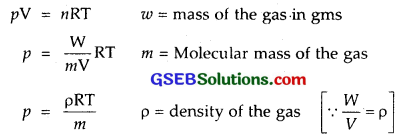Hence density (ρ) of a gas ∝ p [ ∵ RT m are constants]

Question 4.
At 0°C, the density of a certain oxide of a gas at 2 bar is same as that of nitrogen at 5 bar. What is the molecular mass of the oxide ?
Solution:
Density of nitrogen, ρ = $$\frac { Pm }{ RT }$$
where, p = Pressure, m = Molecular mass, R = Gas constant, T = Temperature
Now, P = 5 x 0.987 atm [∵ 1 bar = 0.987 atm]
m = 28, R = 0.0821 L atm/K/mol.
∴ ρ = $$\frac{5 \times 0.987 \times 28}{0.0821 \times 273}$$ [∵ T = 0°C + 273 = 273 K]
Because density of the gaseous oxide is the same
∴ $$\frac{5 \times 0.987 \times 28}{0.0821 \times 273}$$ = $$\frac{2 \times 0.987 \times x}{0.0821 \times 273}$$
∴ x = $$\frac { 5×28 }{ 2 }$$ = 70 g mol-1
∴ Molecular mass of the oxide = 70.0 g mol-1

Question 5.
Pressure of 1 g of an ideal gas A at 27°C is found to be 2 bar. When 2 g of another ideal gas B is introduced in the same flask at same temperature the pressure becomes 3 bar. Find a relationship between their molecular masses.
Solution:
Pressure of 1 gm of an ideal gas A at 27°C (300 K) = 2 bar Pressure on introducing 2 gm of another ideal gas ‘B’ in the same flask = 3 bar
PA = 2 bar; PB = 3 – 2 = 1 bar
Let molecular masses of A and B be MA and BB respectively.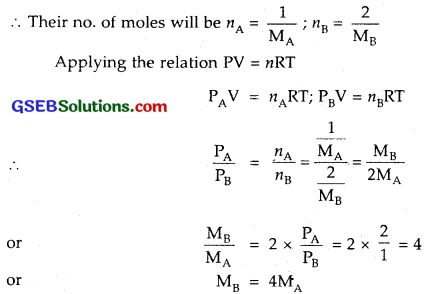Question 6.
The drain cleaner, Drainex contains small bits of aluminium which react with caustic soda to produce dihydrogen. What volume of dihydrogen at 20°C and one bar will be released when 0.15 g of aluminium reacts?
Solution:
The reaction is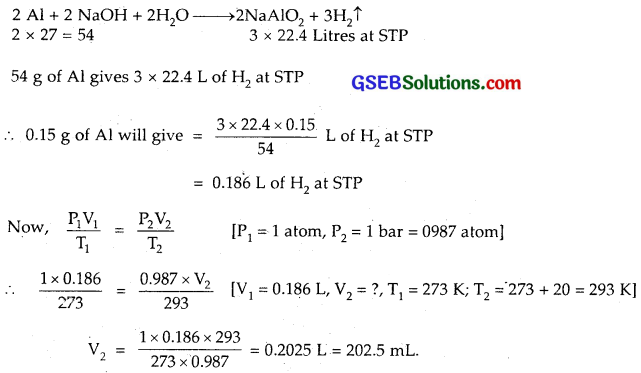Volume of H2 released = 202.5 mL.

Question 7.
What will be the pressure exerted by a mixture of 3.2 g of methane and.4.4 g of carbon dioxide contained in a 9 dm³ flask at 27°C ?
Solution:
Molar mass of methane (CH4) = 12 + 4×1 = 16 g mol-1
Mass of carbon dioxide (CO2) = 12 + 2 x 16 = 44 g mol-1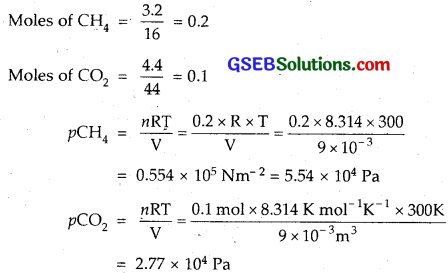Total pressure of mixture
= pCH4 + pCO2 = (5.54 + 2.77) 104 Pa
= 8.32 x 104 Pa.Question 8.
What will be the pressure of the gaseous mixture when 0.5 L of H2 at 0.8 bar and 2.0 L of oxygen at 0.7 bar are introduced in a 1 L vessel at 27°C ?
Solution:
To calculate the partial pressure of H2, i.e., pH2
V1 = 0.5 L V2 = 1L
P1 = 0.8 bar P2 = ?
Temperature remaining constant, applying Boyle’s Law
P1 V1 = p2 V2
0.8 x 0.5 = P2 x 1 or P2 = 0.4 bar
To calculate the partial pressure of O2 i.e., pO2
V1 = 2.0 L V2 = 1 L
P1 = 0.7 bar P2 = ?
Applying Boyle’s Law
p1V1 = P2V2
0.7 x 2.0 = 1 x P2
or P2 = 1.4 bar
If P1 is final pressure of the gas mixture, then according to Dalton’s Law of partial pressures
P = pH2 + pO2 = (0.4 + 1.40) bar = 1.80 bar.

Question 9.
Density of a gas is found to be 5.46 g/dm³ at 27°C at 2 bar pressure. What will be its density at STP?
Solution: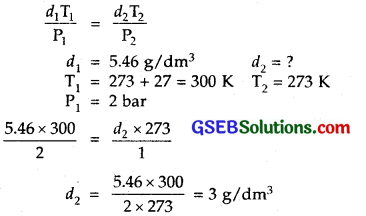Density of the gas at STP = 3 g/dm³.

Question 10.
34.05 mL of phosphorus vapour weighs 0.0625 g at 546°C and 0.1 bar pressure. What is the molar mass of phosphorus?
Solution:
P = 0.1 bar
V = 34.05 mL = 0.03405 dm³
R = 0.083 bar dm³K-1 mol-1
T = 546 + 273 = 819 K
Now PV = nRT
or n = $$\frac { PV }{ RT }$$ = $$\frac{0.1 \times 0.03405}{0.083 \times 819}$$ = 0.00005 mol-1
∴ Molar mass of phosphorus = $$\frac { 0.0625 }{ 0.00005 }$$ = 1250 g mol-1.

Question 11.
A student forgot to add the reaction mixture to the round bottomed flask at 27°C but instead he/she placed the flask on the flame. After a lapse of time, he realized his mistake, and using a pyrometer he found the temperature of the flask was 477°C. What fraction of air would have been expelled out ?
Solution:
T1 = 27 + 273 = 300 K, T2 = 477 + 273 = 750 K
Applying Charles Law :
$$\frac{\mathrm{V}_{1}}{\mathrm{~T}_{1}}$$ = $$\frac{\mathrm{V}_{2}}{\mathrm{~T}_{2}}$$ [As volume remains same]
or $$\frac{\mathrm{V}_{1}}{\mathrm{V}_{2}}$$ = $$\frac { 300 }{ 750 }$$ = $$\frac { 2 }{ 5 }$$
Thus fraction of air expelled = 1 – $$\frac { 2 }{ 5 }$$ = $$\frac { 3 }{ 5 }$$ = 0.6.Question 12.
Calculate the temperature of 4.0 mol of a gas occupying 5 dm³ at 3.32 bar.
Solution:
P = 3.32 bar
V = 5 dm³, R = 0.083 bar dm³ K-1mol-1
n = 4 moles
Since
PV = nRT
T = $$\frac { PV }{ nR }$$
= $$\frac{3.32 \times 5}{4 \times 0.083}$$
= $$\frac { 16.6 }{ 0.332 }$$
= 50 K.

Question 13.
Calculate the total number of electrons present in 1.4 g of nitrogen gas.
Solution:
28 g of N2 gas = 1 mole
1.4 g of N2 gas = $$\frac { 1.4 }{ 28 }$$ = $$\frac { 1 }{ 20 }$$ mole
Total no. of electrons present in $$\frac { 1 }{ 20 }$$ or 0.05 mol of N2
= 0.5 x 6.02 x 1023 x 14 electrons
= 4.2 x 1023 electrons          [ ∵ N2 molecule contains 14 electrons]Question 14.
How much time would it take to distribute one Avogadro number of wheat grains, in 1010 grains are distributed each second?
Solution:
1010 grains are distributed in 1 second
∴ 6.02 x 1023 grains are distributed in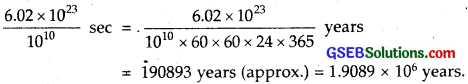Question 15.
Calculate the total pressure in a mixture of 8 g of dioxygen and 4 g of dihydrogen confined in a vessel of 1 dm³ at 27°C R = 0.0833 bar dm³ K-1mol-1.
Solution:
Wt. of O2 = 8.0 gm; Moles of O2 = $$\frac { 8 }{ 32 }$$ = $$\frac { 1 }{ 4 }$$
Wt. of H2 = 4.0 gm; Moles of H2 = $$\frac { 4 }{ 2 }$$ = 2
∴ Total moles present in the mixture = $$\frac { 1 }{ 4 }$$ + 2 = $$\frac { 9 }{ 4}$$
Volume of the vessel = 1 dm³
Now PV = $$\frac { nRT }{ V }$$ = $$\frac { 9 }{ 4 }$$ x $$\frac { 0.083×300 }{ 1 }$$ = 56.025 bar.

Question 16.
Pay load is defned asthe difference between the mass of displaced air and the mass of the balloon. Calculate the pay load when a balloon of radius 10 m, mass loo kg is filled with helium at 1.66 bar at 27°C. (Density of air = 1.2 kg m-3 and R = 0.083 bar dm³ K-1 mol-1).
Solution:
Volume of balloon, V = $$\frac { 4 }{ 3 }$$πr³
Radius of the balloon = 10 m
∴Volume of the balloon = $$\frac { 4 }{ 3 }$$πr³ = $$\frac { 4 }{ 3 }$$ x $$\frac { 22 }{ 7 }$$ (10 m)³
= 4190.5 m³
Volume of the filled at 1.66 bar and 27°C = 4190.5 m³
Calculation of mass of He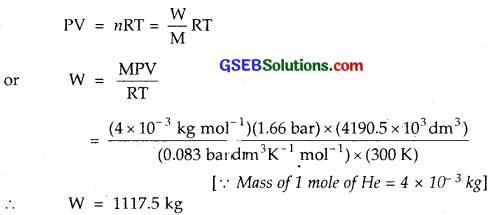Total mass of balloon loaded with He
= 100 + 1117.5 = 1217.5 kg
Maximum mass of the air that can be displaced by balloon to go = Volume x density
= 4190.5 m³ x 1.2 kg m-3 = 5028.6 kg
∴Payload = (5028.6 – 1217.5) kg = 3811.1 kg.Question 17.
Calculate the volume occupied by 8.8 g of CO2 at 31.1°C and 1 bar pressure R = 0.083 bar LK-1 mol-1.
Solution:
P = 1 bar, T = 31.1 + 273 = 304.1 K,
$$\mathrm{W}_{\mathrm{CO}_{2}}$$ = 8.8 g, $$\mathrm{M}_{\mathrm{CO}_{2}}$$ = 44
∴ PV = $$\frac { W }{ m }$$RT
1 x V = $$\frac { 8.8 }{ 44 }$$ x 0.083 x 304.1
or V = 5.05 L.

Question 18.
2.9 g of a gas at 95°C occupied the same volume as 0.184 g of dihydrogen at 17°C, at the same pressure. What is the molarmass of the gas?
Solution:
Applying the ideal gas equation PV = nRT
Case I. Let the molar mass of air = M g mol-1
Mass of gas = 2.9 g
No. of moles m = $$\frac{\text { Mass }}{\text { Molar mass }}$$ = $$\frac { 2.9 }{ M }$$ mol
T = 273 + 95 = 368 K
PV = $$\frac { 2.9 }{ M }$$ x R x 368 … (1)
Case II. Mass of hydrogen = 0.184 g
No. of Moles of H2 = $$\frac { 0.184 }{ 2 }$$
T = 273 + 17 = 290 K
PV = $$\frac { 0.184 }{ 2 }$$ x R x 290 … (2)
From (1) and (2)
$$\frac { 2.9 }{ M }$$ x 368 = $$\frac { 0.184 }{ 2 }$$ x 290
M = $$\frac{2.9 \times 368 \times 2}{0.184 \times 290}$$ = 400 g mol-1

Question 19.
A mixture of dihydrogen and dioxygen at . one bar pressure contains 20% by weight of dihydrogen. Calculate the partial pressure of dihydrogen.
Solution:
If mass of H2 = 20 g, than O2 = 80 g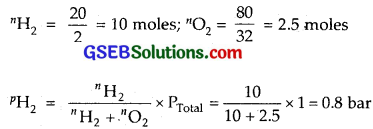Question 20.
What would be the SI unit for the quantity pV²T²/n?
Solution: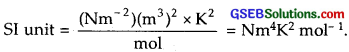Question 21.
In terms of Charles’ law explain why -273°c is the lowest possible temperature.
Solution:
According to Charle’s Law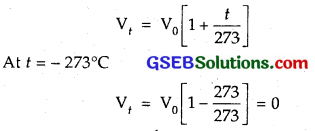Thus, – 273° C is the lowest temperature for a gas because below this temperature, its volume will become negative. This is meaningless.Question 22.
Critical temperature for carbon dioxide and methane are 31.1°C and – 81.9°C respectively. Which of these has stronger intermolecular forces and why?
Solution:
Higher the critical temperature more easily the gas can be liquified, that is, greater are the intermolecular forces of attraction. Hence CO2 has stronger intermolecular forces than CH4.

Question 23.
Explain the physical significance of van der Waals parameters.
Solution:
Significance of constant ‘b’. The constant ‘V is called co-volume or excluded volume per mol of a gas. Its units are litre mol-1. The volume of ‘b’ is four times the actual Volume of the molecules It is measure of effective size of the gas molecules.

Significance of constant ‘a’. The value of constant ‘a’ is a measure of the magnitude of intermolecular forces between the molecules of the gas. Its units are atm L² mol-2. Larger the value of V larger will be the intermolecular forces among the gas molecules.